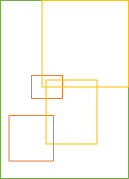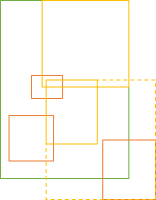A semigroup example for object-oriented programmers.

### Shapes #

Imagine that you're developing a library of two-dimensional shapes, and that, for various reasons, each shape should have a bounding box. For example, here's a blue circle with a green bounding box:The code for a circle shape could look like this:

```public class Circle : ICanHasBox
{
public int X { get; }
public int Y { get; }
public int Radius { get; }

public Circle(int x, int y, int radius)
{
this.X = x;
this.Y = y;
}

public BoundingBox BoundingBox
{
get
{
return new BoundingBox(
}
}
}```

In addition to the `Circle` class, you could have other shapes, such as rectangles, triangles, or even irregular shapes, each of which have a bounding box.

### Bounding box unions #

If you have two shapes, you also have two (green) bounding boxes, but perhaps you'd like to find the (orange) bounding box of the union of both shapes.That's fairly easy to do:

```public BoundingBox Unite(BoundingBox other)
{
var newX = Math.Min(this.X, other.X);
var newY = Math.Min(this.Y, other.Y);

var newRightX = Math.Max(this.rightX, other.rightX);
var newTopY = Math.Max(this.topY, other.topY);

return new BoundingBox(
newX,
newY,
newRightX - newX,
newTopY - newY);
}```

The `Unite` method is an instance method on the `BoundingBox` class, so it's a binary operation. It's also associative, because for all `x`, `y`, and `z`, `isAssociative` is `true`:

`var isAssociative = x.Unite(y).Unite(z) == x.Unite(y.Unite(z));`

Since the operation is associative, it's at least a semigroup.

### Lack of identity #

Is `Unite` also a monoid? In order to be a monoid, a binary operation must not only be associative, but also have an identity element. In a previous article, you saw how the union of two convex hulls formed a monoid. A bounding box seems to be conceptually similar to a convex hull, so you'd be excused to think that our previous experience applies here as well.

It doesn't.

There's no identity bounding box. The difference between a convex hull and a bounding box is that it's possible to define an empty hull as an empty set of coordinates. A bounding box, on the other hand, always has a coordinate and a size.

```public struct BoundingBox
{

public int X { get; }
public int Y { get; }
public int Width { get; }
public int Height { get; }

// More members, including Unite...
}```

An identity element, if one exists, is one where if you `Unite` it with another `BoundingBox` object, the return value will be the other object.

Consider, then, a (green) `BoundingBox x`. Any other `BoundingBox` inside of `x`, including `x` itself, is a candidate for an identity element:In a real coordinate system, there's infinitely many candidates contained in `x`. As long as a candidate is wholly contained within `x`, then the union of the candidate and `x` will return `x`.

In the code example, however, coordinates are 32-bit integers, so for any bounding box `x`, there's only a finite number of candidates. Even for the smallest possible bounding box, though, the box itself is an identity candidate.

In order to be an identity element, however, the same element must behave as the identity element for all bounding boxes. It is, therefore, trivial to find a counter-example:Just pick any other `BoundingBox y` outside of `x`. Every identity candidate must be within `x`, and therefore the union of the candidate and `y` cannot be `y`.

In code, you can demonstrate the lack of identity with an FsCheck-based test like this:

```[Property(QuietOnSuccess = true)]
public Property UniteHasNoIdentity(PositiveInt w, PositiveInt h)
{
var genCandidate = from xi in Gen.Choose(1, w.Get)
from yi in Gen.Choose(1, h.Get)
from wi in Gen.Choose(1, w.Get - xi + 1)
from hi in Gen.Choose(1, h.Get - yi + 1)
select new BoundingBox(xi, yi, wi, hi);
return Prop.ForAll(
genCandidate.ToArbitrary(),
identityCandidate =>
{
var x = new BoundingBox(1, 1, w.Get, h.Get);

// Show that the candidate behaves like identity for x
Assert.Equal(x, x.Unite(identityCandidate));
Assert.Equal(x, identityCandidate.Unite(x));

// Counter-example
var y = new BoundingBox(0, 0, 1, 1);
Assert.NotEqual(y, y.Unite(identityCandidate));
});
}```

This example uses the FsCheck.Xunit glue library for xUnit.net. Notice that although FsCheck is written in F#, you can also use it from C#. This test passes.

It follows the above 'proof' by first generating an identity candidate for `x`. This is any `BoundingBox` contained within `x`, including `x` itself. In order to keep the code as simple as possible, `x` is always placed at the coordinate (1, 1).

The test proceeds to utilise two Guard Assertions to show that `identityCandidate` does, indeed, behave like an identity for `x`.

Finally, the test finds a trivial counter-example in `y`, and verifies that `y.Unite(identityCandidate)` is not equal to `y`. Therefore, `identityCandidate` is not the identity for `y`.

`Unite` is a semigroup, but not a monoid, because no identity element exists.

### Summary #

This article demonstrates (via an example) that non-trivial semigroups exist in normal object-oriented programming.

Next: Semigroups accumulate.

Thank you for writing about category theory. I just have a small note. "Just pick any other BoundingBox y partially or wholly outside of x." I think that one should pick a BoundingBox y wholly outside of x. Other wise the intersection between x and y would return x or y when we pass it to x.Unite or y.Unite respectively.
Thanks
2017-12-08 16:04 UTC

Yacoub, thank you for writing. The operation used here isn't the intersection, but rather the union of two bounding boxes; that's the reason I called the method `Unite`.

2017-12-09 12:55 UTC

Hello Mark. I am aware of this, but maybe I did not explain my self correctly.
What I am trying to say is that when coming up with a counter-example, we should choose a BoundingBox y wholly outside of x (not just partially outside of x).
If we choose a BoundingBox y partially outside of x, then the intersection between x and y (the BoundingBox z = the area shared between x and y) is a valid identity element.

2017-12-09 13:05 UTC

Yacoub, I think you're right; sorry about that!

Perhaps I should have written Just pick any other `BoundingBox y` partially or wholly outside of the candidate. Would that have been correct?

2017-12-09 13:57 UTC

That would be correct. I am not sure though if this is the best way to explain it.

y being wholly ourside of x seems better to me.

2017-12-09 14:15 UTC

Yacoub, I've corrected the text in the article. Thank you for the feedback!

2017-12-09 15:21 UTC

### Wish to comment?

You can add a comment to this post by sending me a pull request. Alternatively, you can discuss this post on Twitter or somewhere else with a permalink. Ping me with the link, and I may respond.

#### Published

Monday, 04 December 2017 08:40:00 UTC

#### Tags

"Our team wholeheartedly endorses Mark. His expert service provides tremendous value."
Hire me!
Published: Monday, 04 December 2017 08:40:00 UTC